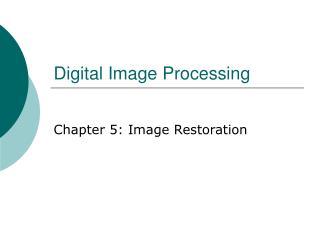Download PresentationDigital Image Processing

Digital Image Processing - PowerPoint PPT Presentation

Digital Image Processing. Chapter 5: Image Restoration. A Model of the Image Degradation/Restoration Process. Degradation Degradation function H Additive noise Spatial domain Frequency domain. Restoration. Noise Models. Sources of noise Image acquisition, digitization, transmissionI am the owner, or an agent authorized to act on behalf of the owner, of the copyrighted work described.
Download PresentationDigital Image Processing

Download Policy: Content on the Website is provided to you AS IS for your information and personal use and may not be sold / licensed / shared on other websites without getting consent from its author.While downloading, if for some reason you are not able to download a presentation, the publisher may have deleted the file from their server.

- - - - - - - - - - - - - - - - - - - - - - - - - - E N D - - - - - - - - - - - - - - - - - - - - - - - - - -
Presentation Transcript
1. Digital Image Processing Chapter 5: Image Restoration

2. A Model of the Image Degradation/Restoration Process

4. Restoration

5. Noise Models • Sources of noise • Image acquisition, digitization, transmission • White noise • The Fourier spectrum of noise is constant • Assuming • Noise is independent of spatial coordinates • Noise is uncorrelated with respect to the image itself

6. Gaussian noise • The PDF of a Gaussian random variable, z, • Mean: • Standard deviation: • Variance:

7. 70% of its values will be in the range • 95% of its values will be in the range

8. Rayleigh noise • The PDF of Rayleigh noise, • Mean: • Variance:

9. Erlang (Gamma) noise • The PDF of Erlang noise, , is a positive integer, • Mean: • Variance:

10. Exponential noise • The PDF of exponential noise, , • Mean: • Variance:

11. Uniform noise • The PDF of uniform noise, • Mean: • Variance:

12. Impulse (salt-and-pepper) noise • The PDF of (bipolar) impulse noise, • : gray-level will appear as a light dot, while level will appear like a dark dot • Unipolar: either or is zero

13. Modeling • Gaussian • Electronic circuit noise, sensor noise due to poor illumination and/or high temperature • Rayleigh • Range imaging • Exponential and gamma • Laser imaging

14. Impulse • Quick transients, such as faulty switching • Uniform • Least descriptive • Basis for numerous random number generators

15. Periodic noise • Arises typically from electrical or electromechanical interference • Reduced significantly via frequency domain filtering

16. Estimation of noise parameters • Inspection of the Fourier spectrum • Small patches of reasonably constant gray level • For example, 150*20 vertical strips • Calculate , , , from

17. Restoration in the Presence of Noise Only-Spatial Filtering • Degradation • Spatial domain • Frequency domain

18. Mean filters • Arithmetic mean filter • Geometric mean filter

19. Harmonic mean filter • Works well for salt noise, but fails fpr pepper noise

20. Contraharmonic mean filter • : eliminates pepper noise • : eliminates salt noise

21. Usage • Arithmetic and geometric mean filters: suited for Gaussian or uniform noise • Contraharmonic filters: suited for impulse noise

22. Order-statistics filters • Median filter • Effective in the presence of both bipolar and unipolar impulse noise

23. Max and min filters • max filters reduce pepper noise • min filters salt noise

24. Midpoint filter • Works best for randomly distributed noise, like Gaussian or uniform noise

25. Alpha-trimmed mean filter • Delete the d/2 lowest and the d/2 highest gray-level values • Useful in situations involving multiple types of noise, such as a combination of salt-and-pepper and Gaussian noise

26. Adaptive, local noise reduction filter • If is zero, return simply the value of • If , return a value close to • If , return the arithmetic mean value

27. Adaptive median filter • = minimum gray level value in • = maximum gray level value in • = median of gray levels in • = gray level at coordinates • = maximum allowed size of

28. Algorithm: • Level A: A1= • A2= • If A1>0 AND A2<0, Go to • level B • Else increase the window size • If window size • repeat level A • Else output

29. Level B: B1= • B2= • If B1>0 AND B2<0, output • Else output

30. Purposes of the algorithm • Remove salt-and-pepper (impulse) noise • Provide smoothing • Reduce distortion, such as excessive thinning or thickening of object boundaries

31. Periodic Noise Reduction by Frequency Domain Filtering • Bandreject filters • Ideal bandreject filter

32. Butterworth bandreject filter of order n • Gaussian bandreject filter

33. Bandpass filters